• ### get phase of [osc~]

[phasor~]
|
[cos~]

should be the same as
[osc~]

but this time I need to measure the phase of [osc~]
and do the same as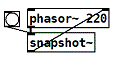I have a running patch, but sometimes it flips the phase by 180 degrees.
Do you know why or how to avoid that?

Here is the patch:
phase_of_normalized_cosine~.pd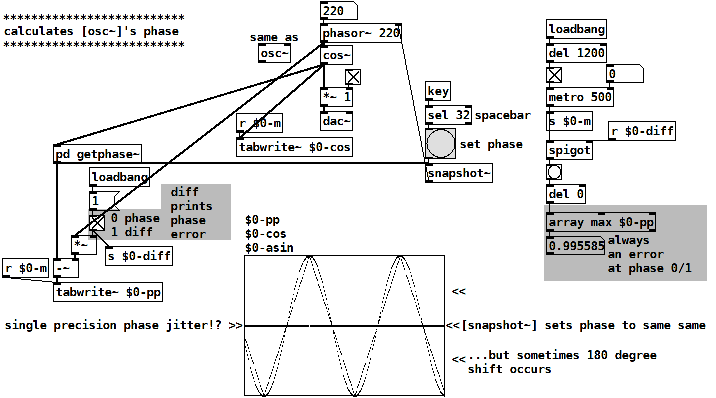The patch calculates the phase of a normalized cosine y(x) by
asin(y(x)) / (pi/2)
this is a triangle wave, following cosine's shape linearized.
Then a tri-to-saw waveshper:
invert the falling part of the triangle
and divide it's frequency by 2.

• Posts 9 | Views 765
• @lacuna Fun problem, thanks! I haven't understood your patch completely, but maybe try using this instead of your getphase~ subpatch and see if things work any better. Maybe you have a 1 sample delay somewhere?
norm cosine to phasor.pd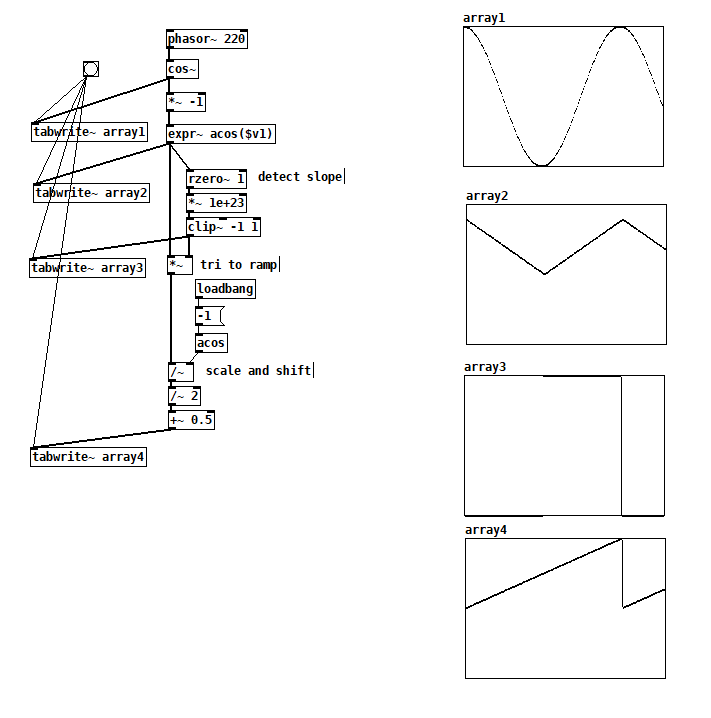Edit: Oh OK, I'm getting an occasional "phase shift" at the 1-0 transition too, but that's a ~360 degree phase shift, not 180 degrees, i.e. it doesn't matter WRT cos~.

• Thank you very much @jameslo
this patch means something else to me!!!

Your tri to ramp conversion by mirroring the falling part of the triangle by *~ -1 seems to be the definitive answer and by the screenshot I do understand visually.

Yes, it must have been the sh-flip-flop in my patch, somehow flipping or flopping one way or another around, - only wondering why that is not the case on your machine, here a 180° shift happens a lot actually.

Anyway...
Thank you very very much!

EDIT:• @lacuna Actually, I think it might have been happening on my machine, but I edited that comment away when I realized how poorly I understood your patch• At risk of missing the point, but...

Pretty much every programming problem boils down to these three questions:

1. What information do I have?
2. What information do I want to have?
3. What operations will get me from 1 to 2?
``````[phasor~]
|
[cos~]
``````

If we are starting here, then question 1 is "I have a phase, and a cosine."

Question 2 is "I want the phase."

Sooooooo... the Occam's Razor solution is not to discard the phase and recalculate it trigonometrically, but rather simply to use the phasor~ signal.

The mathematical problem that jameslo dealt with is that every value -1 < cosx < 1 occurs twice within a cycle. You can use the acos() angle directly if the cosx signal is falling (negative slope), but if it's rising, you must negate the angle.

But I think my practical point remains. If you need the phase, you can get the phase and the sinusoid using two objects, and have both signals available to do whatever you need. jameslo's solution involves 9 signal-rate objects. Eliminating the [phasor~] at the top ends up adding A LOT more complexity (more chances for bugs etc) and at a certain point (while acknowledging the appeal of intellectual curiosity), it stops being worth it.

The simplest solution that achieves the result is usually the best.

hjh

• Though I agree that it's better to get the phase in another way if you can, there is a simple way to do this if you really have to.

The phase output works only on pure sine waves, if you use anything else, it will look distorted.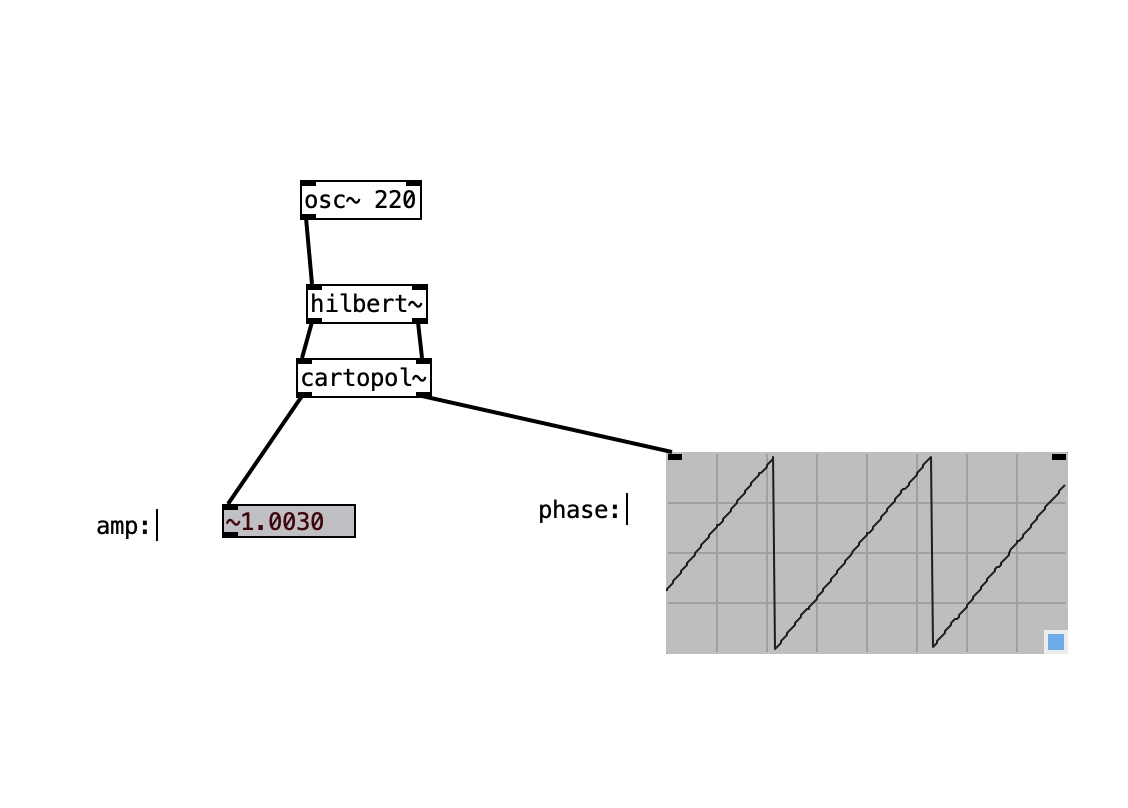This works by phase-shifting the signal 90°, and looking at the plot of the two signals (one on x-axis, one on y-axis). The rotation represents phase, the radius represents the amplitude.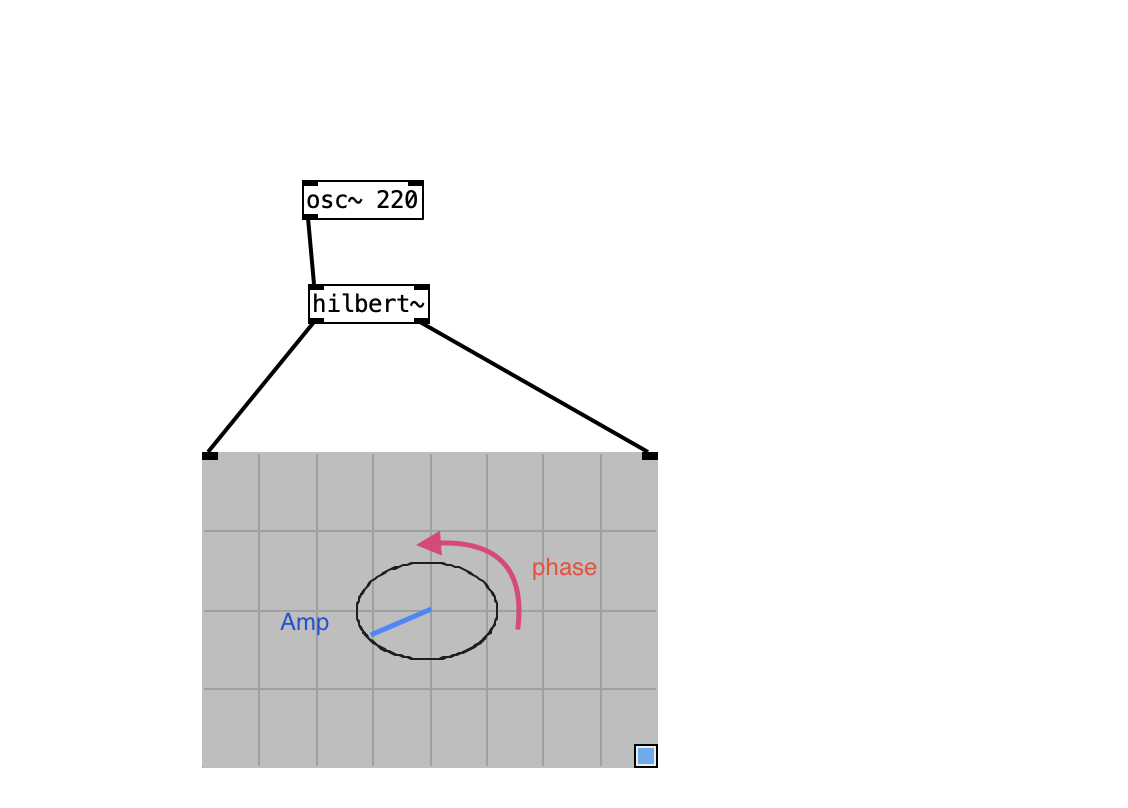• @timothyschoen My understanding is that hilbert~ is implemented with two all-pass filters, so while the phase between the outputs is approx pi/2, the phase between the input and either output varies by frequency. Were that not the case, the following test would output a constant close to 1.
norm cosine to phasor using hilbert.pd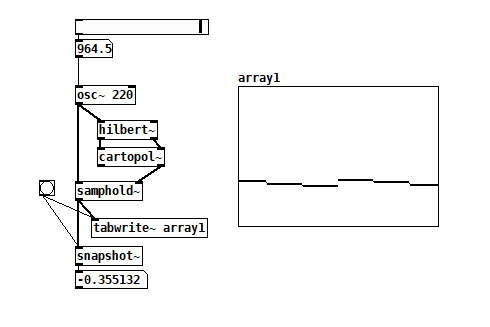I don't think that's what @lacuna is asking for.

• @jameslo Good point, I didn't consider that! You could use the real output of the [hilbert~] instead of the [osc~], but at that point it's not really a solution to this problem anymore. Thanks for pointing this out.

• @timothyschoen You got me really excited though! ThanksPosts 9 | Views 765
Internal error.

Oops! Looks like something went wrong!